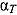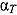# Analytical, Semi-Analytical, and Numerical Heavy-Gas Verification Benchmarks of the Effective Multiplication Factor and Temperature Coefficient

Nuclear Science and Engineering / Volume 191 / Number 1 / July 2018 / Pages 1-45

Technical Paper / dx.doi.org/10.1080/00295639.2018.1442546

The heavy-gas model with specific energy-dependent absorption cross sections is used to construct analytical, semi-analytical, and numerical free-gas scattering benchmarks for the neutron spectrum, effective multiplication factor k, and temperature coefficientin an infinite, homogeneous medium. The energy dependences considered are piecewise constant, constant plus inverse in energy, and piecewise linear. Analytic forms for k andin terms of hypergeometric functions are obtained for piecewise-constant absorption with two energy ranges and for constant-plus-inverse-in-energy absorption. Analogous semi-analytical integral expressions are obtained for piecewise-linear absorption with two energy ranges. Numerical solutions of a linear system are obtained for piecewise-constant and piecewise-linear absorption for greater than two energy ranges. The heavy-gas model solutions of k are compared with continuous-energy Monte Carlo calculations; the results converge to the heavy-gas model with increasing target mass ratio A, demonstrating the heavy-gas model’s utility as a verification benchmark.